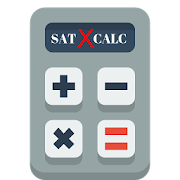sat maths without calculatorEveryone
2
sat maths without calculator app provide free model practice tests covered all types of maths problems preparation with solution.

SAT Exam Math Test Overview

The Math Test has been redesigned to include two portions: one using a calculator, and one without. Both portions of the test focus in depth on the three areas of math most widely used in most college majors and careers: algebra, problem solving and data analysis, and manipulations of complex equations. Besides these three major areas, the Math Test will also test students’ knowledge of geometry, trigonometry, and common theorems or formulas.

While most of the questions are multiple choice, the Math Test contains 17 grid-in questions. Instead of choosing from a pre-provided list of possible answers, students must solve the problem and then enter the answer in the grid provided on their answer sheet.

Tips to solve SAT MATH without Calculator Tests

1] The No-Calculator Math Test is the third multiple-choice section of the SAT. You will have 25 minutes to answer 15 multiple choice questions and 5 grid-in questions, leaving you with a little more than a minute per question.

2] You are likely accustomed to solving a question, arriving at an answer, and crossing your fingers, hoping it matches one of the choices given. However, as 15 of the 20 no-calculator questions are multiple-choice, you may find that working backwards is helpful in sat maths without calculator.

3] When multiple-choice questions on the SAT provide expressions, graphs, or phrases with variables, you may be able to pick numbers, which will make these questions both easier to understand and to solve.

4] The No-Calculator Math questions will not require long, drawn-out calculations. Remember: these questions are all designed to take less than a minute to solve. Look for patterns and shortcuts to solve complicated-looking questions more easily.

5] The No-Calculator Math Test will require you to solve a lot of equations by hand. Make sure you are familiar with all of the following equation-solving techniques:

=> Cardinal rule of equations—do the same thing to both sides of the equation. For example, if you divide the left side of the equation by 3, divide the right side of the equation by 3 as well.

=> Clearing fractions—when an equation includes lots of fractions, find the lowest common denominator (LCD) of all fractions, and multiply the entire equation by this LCD. This will eliminate fractions from your equation and make it much easier to solve in sat maths without calculator.

=> Solve a system of two equations with two variables—know how to use both substitution (solve for an equation in terms of one variable and plug it into the other) and combination/elimination (multiply both sides of one equation by a number that will allow you to eliminate a variable when you add the two equations together).
Cross multiplying—when you have an equation with a single fraction on each side, multiply the denominator of the left side by the numerator of the right and vice-versa. Set these two products equal to each other to get a more straightforward equation to solve.

=> Factor and solve quadratics—to solve a quadratic equation, you must first get it to the form ax2 + bx + c = 0, then factor, and finally set each factor equal to 0. If an equation is not easily factored, you can use the quadratic formula:
Collapse

Review Policy
3.0
2 total
5
4
3
2
1

Updated
October 16, 2018
Size
1.8M
Installs
500+
Current Version
2.0
Requires Android
4.0.3 and up
Content Rating
Everyone
Permissions
Offered By
Examguide
Developer